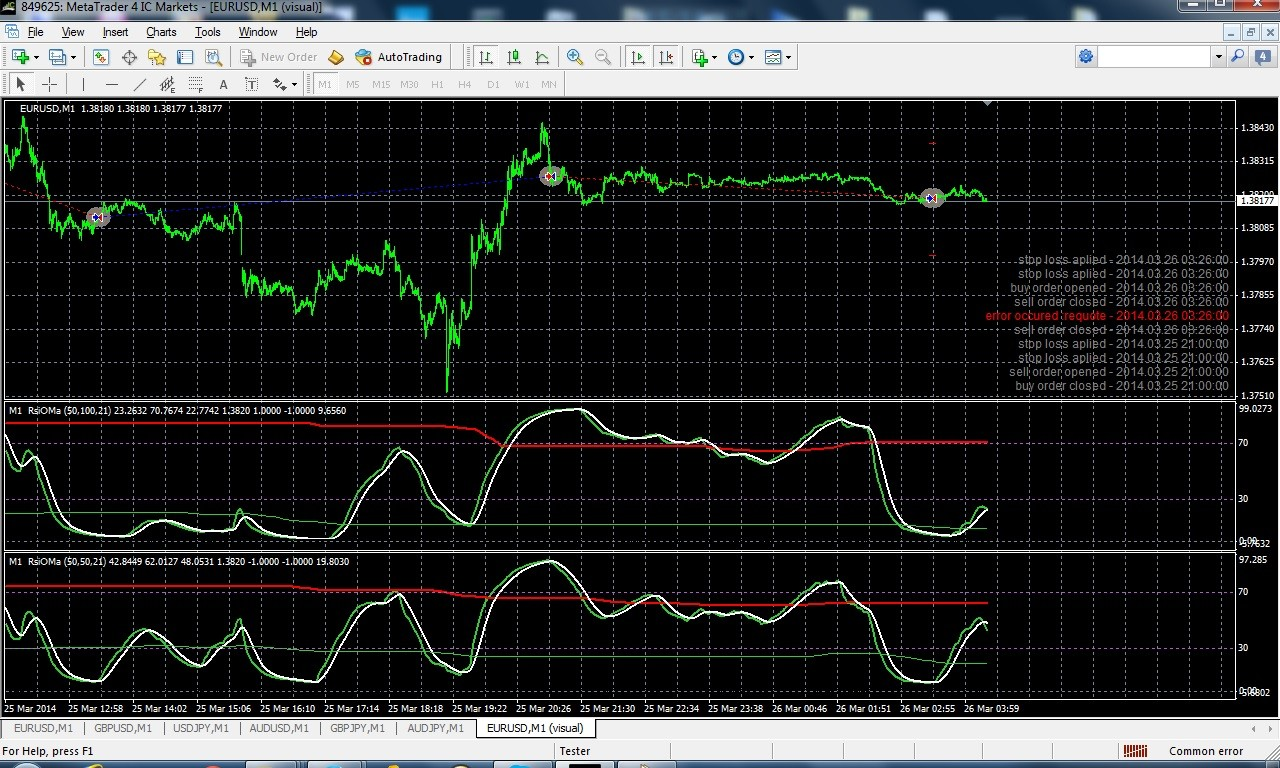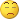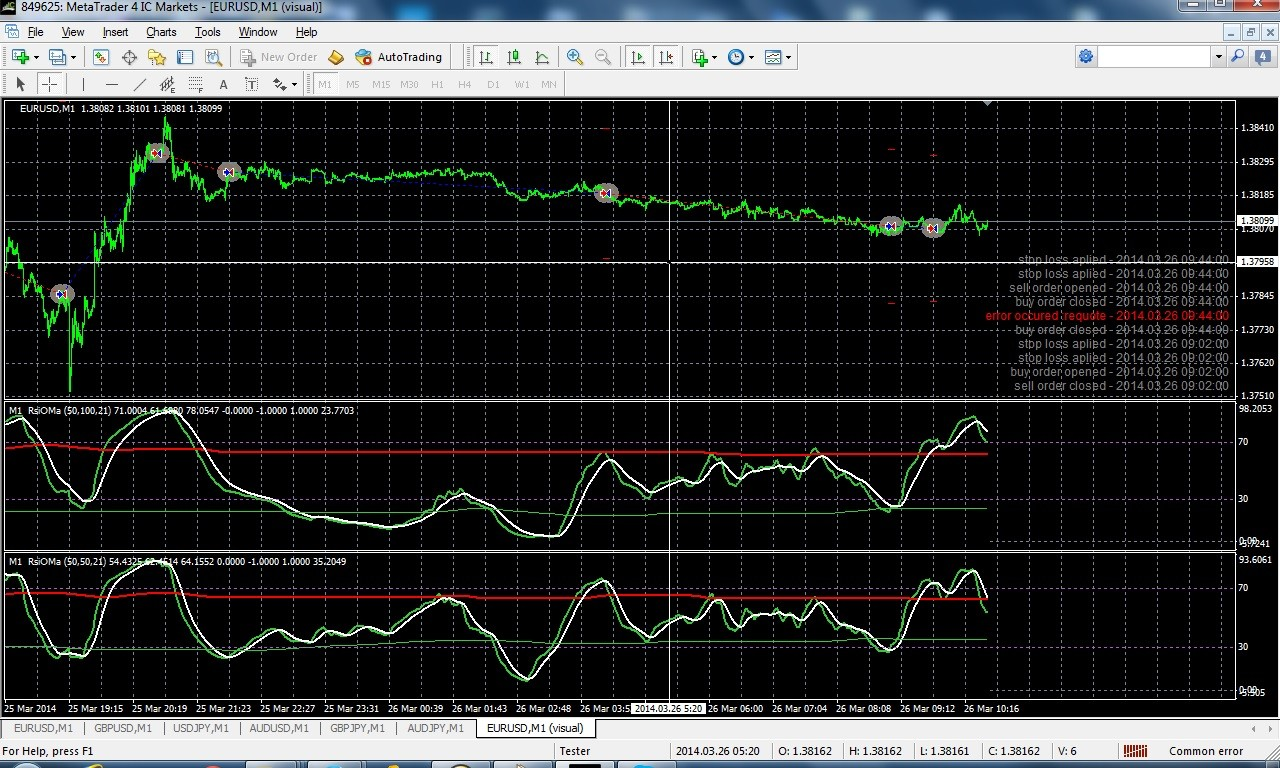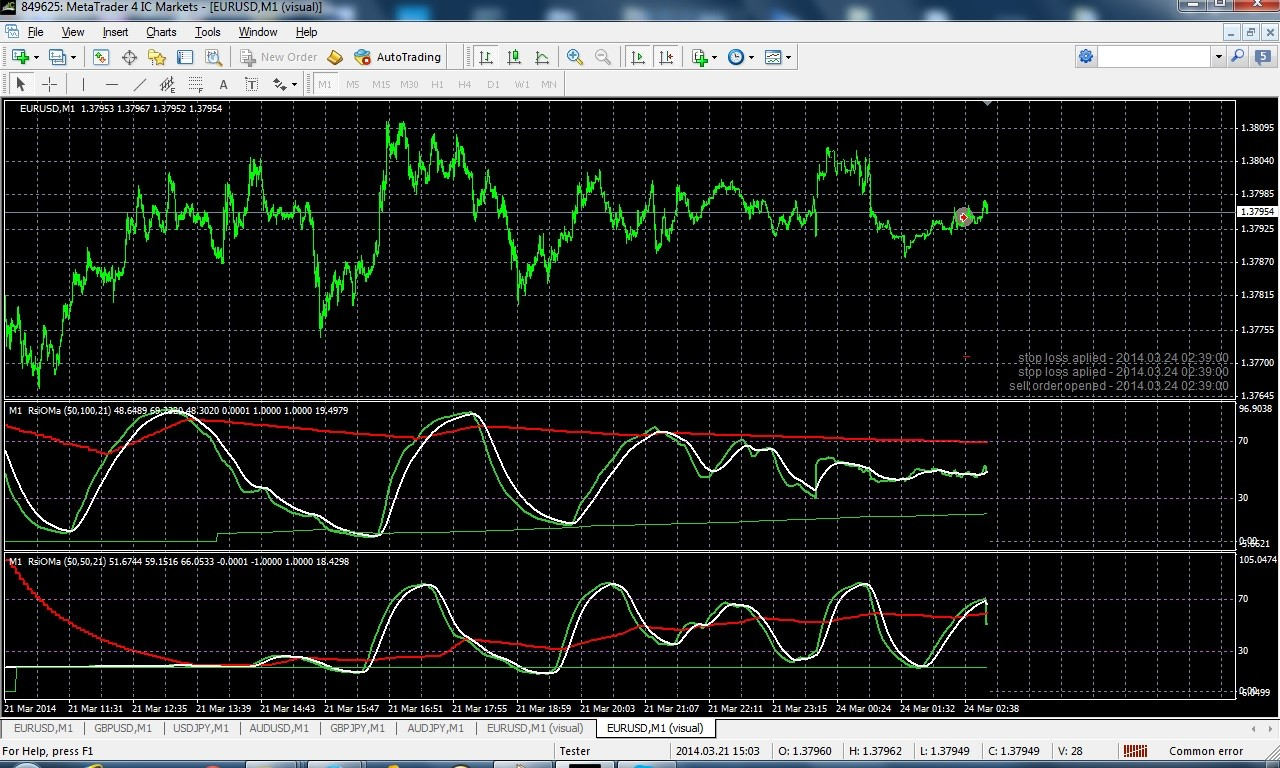# RSI of Moving Average - page 31156281

fajst_k:
No. In bot cases DZ.... are set to 0.25. Lookback also the same.

extern int DzLookBackBars = 1440;

extern double DzStartSellProbability = 0.25;

Krzysztof

I am not referring to parameter(s). I am referring to the source array of data for calculation that is passed to dz calculation as a basis for calculation (the first parameter that is passed to the functions - the sourceArray[] from the functions prototypes). If (as it is obvious from the displayed chart you posted) the values used for calculation are different, the zones must be different too (regardless of the same parameters).298

Krzysztof I am not referring to parameter(s). I am referring to the source array of data for calculation that is passed to dz calculation as a basis for calculation (the first parameter that is passed to the functions - the sourceArray[] from the functions prototypes). If (as it is obvious from the displayed chart you posted) the values used for calculation are different, the zones must be different too (regardless of the same parameters).

Yes, this what I suspect but there is no black magic here, only difference is that the ticks are generated by MT4 tester

Here is a code. Both RSI and bands calculation seems to be affected by this.

if (calculateValue || timeFrame==Period())

{

if (slope[limit] == -1) ClearPoint(limit,rsida,rsidb);

if (detrend) for (i=limit; i >= 0; i--) mab = iMA(NULL,0,Detrend_Period,0,Before_Mode,Before_Price,i);

if (!detrend)for (i=limit; i >= 0; i--) mab = iMA(NULL,0,Period_Before,0,Before_Mode,Before_Price,i);

if (detrend) {

for (i=limit; i >= 0; i--) mab = Close-mab;

for (i=limit; i >= 0; i--) mab = iMAOnArray(mab,0,Period_Before,0,MODE_SMA,i);

}

for (i=limit; i >= 0; i--) rsi = iRSIOnArray(mab,0,RsiOma_Period,i);

for (i=limit; i >= 0; i--)

{

ma = iMAOnArray(rsi,0,MaPeriod,0,MaType,i);

rsida = EMPTY_VALUE;

rsidb = EMPTY_VALUE;

slope = slope;

trend = trend;

if (rsi > rsi) slope= 1;

if (rsi < rsi) slope=-1;

if (rsi > ma) trend= 1;

if (rsi < ma) trend=-1;

if (slope == -1) PlotPoint(i,rsida,rsidb,rsi);

sli = dzSell(rsi,DzStartSellProbability, DzLookBackBars, Bars, i );

}156281

fajst_k:
Yes, this what I suspect but there is no black magic here, only difference is that the ticks are generated by MT4 tester

Here is a code. Both RSI and bands calculation seems to be affected by this.

if (calculateValue || timeFrame==Period())

{

if (slope[limit] == -1) ClearPoint(limit,rsida,rsidb);

if (detrend) for (i=limit; i >= 0; i--) mab = iMA(NULL,0,Detrend_Period,0,Before_Mode,Before_Price,i);

if (!detrend)for (i=limit; i >= 0; i--) mab = iMA(NULL,0,Period_Before,0,Before_Mode,Before_Price,i);

if (detrend) {

for (i=limit; i >= 0; i--) mab = Close-mab;

for (i=limit; i >= 0; i--) mab = iMAOnArray(mab,0,Period_Before,0,MODE_SMA,i);

}

for (i=limit; i >= 0; i--) rsi = iRSIOnArray(mab,0,RsiOma_Period,i);

for (i=limit; i >= 0; i--)

{

ma = iMAOnArray(rsi,0,MaPeriod,0,MaType,i);

rsida = EMPTY_VALUE;

rsidb = EMPTY_VALUE;

slope = slope;

trend = trend;

if (rsi > rsi) slope= 1;

if (rsi < rsi) slope=-1;

if (rsi > ma) trend= 1;

if (rsi < ma) trend=-1;

if (slope == -1) PlotPoint(i,rsida,rsidb,rsi);

sli = dzSell(rsi,DzStartSellProbability, DzLookBackBars, Bars, i );

}

Krzysztof

MT tester (and the way it generates ticks) is one of the funniest things there is. It has nothing in common with reality (I have posted some picture of ticks generated by tester in the "various" thread - can not find it right now, but what it was showing is a ridiculous generated distribution that was nothing like reality)298

Krzysztof MT tester (and the way it generates ticks) is one of the funniest things there is. It has nothing in common with reality (I have posted some picture of ticks generated by tester in the "various" thread - can not find it right now, but what it was showing is a ridiculous generated distribution that was nothing like reality)

Tick distribution only applies to 'within the bar' time, it should hold OHLC values or ???

Anyway I tried another testing model called 'control point' but it seems to be similar - there

is a mismatch between tester drawn and offline drawn charts.

So what is a conclusion ?? How is possible to back test something having only OHLC values in MT4 and no such mismatch ??Files:
rsioma2.jpg 415 kb298

fajst_k:
Tick distribution only applies to 'within the bar' time, it should hold OHLC values or ???

Anyway I tried another testing model called 'control point' but it seems to be similar - there

is a mismatch between tester drawn and offline drawn charts.

So what is a conclusion ?? How is possible to back test something having only OHLC values in MT4 and no such mismatch ??I tried 3rd model 'open prices' and its the same. All of them give this mismatch156281

fajst_k:
Tick distribution only applies to 'within the bar' time, it should hold OHLC values or ???

Anyway I tried another testing model called 'control point' but it seems to be similar - there

is a mismatch between tester drawn and offline drawn charts.

So what is a conclusion ?? How is possible to back test something having only OHLC values in MT4 and no such mismatch ??:)Welcome to the club

We are "crying wolf" (that metatrader tester is useless) for so long time that people by now think that we are like that kid that was not telling the truth. But, unfortunately, we are (telling the truth).

Whatever method they use for generating data in back-test (they are calling it a "genetic algorithm") you will find out that it makes the same nonsense at the same places over and over, so, nothing with the implied intra-bar randomness of the "genetic algorithm" nor does it honor the obvious what should be honored (the OHLC values). As if it was deliberately made to avoid similarity to realty298

:):)Welcome to the club

We are "crying wolf" (that metatrader tester is useless) for so long time that people by now think that we are like that kid that was not telling the truth. But, unfortunately, we are (telling the truth).

Whatever method they use for generating data in back-test (they are calling it a "genetic algorithm") you will find out that it makes the same nonsense at the same places over and over, so, nothing with the implied intra-bar randomness of the "genetic algorithm" nor does it honor the obvious what should be honored (the OHLC values). As if it was deliberately made to avoid similarity to realty

I think OHLC is held. I just made another test and if you plot simple RSI mismatch don't occur.

(basic test methodology - if nothing works back to the square 1). So RSI which is implemented

in MT4 as separate script is working.

But i think during 'test mode' some imprecisions are occuring most likely for speeding up as the biggest mismatch occurs for this test in detrended mode as Close-mab is a very small value.

So all those iMAOnArray/iRSIOnArray/iMA internally may use much lower precision than 'double'

Simple test. Rewrite this indi using scripts like SimpleMAOnBuffer from MovingAverages.mqh

but not build in functions would confirm/deny this theory156281

fajst_k:
I think OHLC is held. I just made another test and if you plot simple RSI mismatch don't occur.

(basic test methodology - if nothing works back to the square 1). So RSI which is implemented

in MT4 as separate script is working.

But i think during 'test mode' some imprecisions are occuring most likely for speeding up as the biggest mismatch occurs for this test in detrended mode as Close-mab is a very small value.

So all those iMAOnArray/iRSIOnArray/iMA internally may use much lower precision than 'double'

Simple test. Rewrite this indi using scripts like SimpleMAOnBuffer from MovingAverages.mqh

but not build in functions would confirm/deny this theory

If I remember correctly built in sma has a serious error - it never can reach the value of 0 (even when the sequence of enough zeroes is present in the sample - in which case sma must be equal to 0). Also I think that they have similar problems with smma and lwma. So far I haven't noticed problems only with their built in EMA

Anyway, that is one of the reasons why I prefer to write my own functions - that way I am sure that even if I notice an error in it I can correct it and not just look at it and accept wrong results (and there are quite a few such cases when it comes to metatrader built in functions - unfortunately)298

If I remember correctly built in sma has a serious error - it never can reach the value of 0 (even when the sequence of enough zeroes is present in the sample - in which case sma must be equal to 0). Also I think that they have similar problems with smma and lwma. So far I haven't noticed problems only with their built in EMA Anyway, that is one of the reasons why I prefer to write my own functions - that way I am sure that even if I notice an error in it I can correct it and not just look at it and accept wrong results (and there are quite a few such cases when it comes to metatrader built in functions - unfortunately)

Working !!! iMAOnArray(mab,0,Period_Before,0,MODE_SMA,i); in combination with using of MT4 tester was a problem. I changed everywhere to MODE_EMA and its ok now !!!

See screenshoot in detrend mode when MODE_EMA and MODE_SMA.

So tester is OK most likely....ufff, beautifull day gone on digging on this...

KrzysztofFiles:
working.jpg 414 kb
notworking.jpg 413 kb298

Here is some EA results based on RSIOMA with Dynamic zone probability bands with DZ settings 0.05, 0.1, 0.15 and 0.2

for last week EURUSD 1min. The system generated not so many trades but from results you can see that only for

values 0.05 and 0.1 PF had some reasonable value (0.82). Turning on detrending seems to decrease performance for those settings

Krzysztof

Files:
rsioma.rar 50 kb